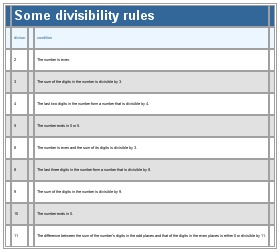# Divisibility rules

Divisibility rules

Table
Some divisibility rules
divisor
condition
2
The number is even.
3
The sum of the digits in the number is divisible by 3.
4
The last two digits in the number form a number that is divisible by 4.
5
The number ends in 0 or 5.
6
The number is even and the sum of its digits is divisible by 3.
8
The last three digits in the number form a number that is divisible by 8.
9
The sum of the digits in the number is divisible by 9.
10
The number ends in 0.
11
The difference between the sum of the number’s digits in the odd places and that of the digits in the even places is either 0 or divisible by 11.
See as table:See as table:* * *

Universalium. 2010.

### Look at other dictionaries:

• Divisibility rule — A divisibility rule is a shorthand way of discovering whether a given number is divisible by a fixed divisor without performing the division, usually by examining its digits. Although there are divisibility tests for numbers in any radix, and… …   Wikipedia

• Divisor — divisible redirects here. For divisibility of groups, see Divisible group. For the second operand of a division, see Division (mathematics). For divisors in algebraic geometry, see Divisor (algebraic geometry). For divisibility in the ring theory …   Wikipedia

• arithmetic — arithmetically, adv. n. /euh rith meuh tik/; adj. /ar ith met ik/, n. 1. the method or process of computation with figures: the most elementary branch of mathematics. 2. Also called higher arithmetic, theoretical arithmetic. the theory of… …   Universalium

• Division (mathematics) — Divided redirects here. For other uses, see Divided (disambiguation). For the digital implementation of mathematical division, see Division (digital). In mathematics, especially in elementary arithmetic, division (÷ …   Wikipedia

• Fermat's Last Theorem in fiction — The famous problem in number theory known as Fermat s Last Theorem has repeatedly received attention in fiction and popular culture.* In The Royale , an episode of , Captain Picard states that the theorem had gone unsolved for 800 years. At the… …   Wikipedia

• Fibonacci number — A tiling with squares whose sides are successive Fibonacci numbers in length …   Wikipedia

• Finitary relation — This article sets out the set theoretic notion of relation. For a more elementary point of view, see Binary relation. For a combinatorial viewpoint, see Theory of relations. For other uses, see Relation (disambiguation). In set theory and logic,… …   Wikipedia

• Parity (mathematics) — In mathematics, the parity of an object states whether it is even or odd. This concept begins with integers. An even number is an integer that is evenly divisible by 2, i.e., divisible by 2 without remainder; an odd number is an integer that is… …   Wikipedia

• Parity of zero — Zero objects, divided into two equal groups Zero is an even number. In other words, its parity the quality of an integer being even or odd is even. Zero fits the definition of even number : it is an integer multiple of 2, namely 0 × 2. As a… …   Wikipedia

• Polynomial — In mathematics, a polynomial (from Greek poly, many and medieval Latin binomium, binomial   , the word has been introduced, in Latin, by Franciscus Vieta) is an expression of finite length constructed from variables (also known as… …   Wikipedia